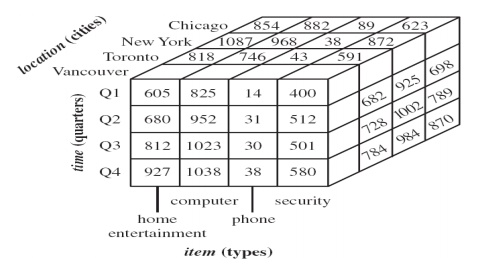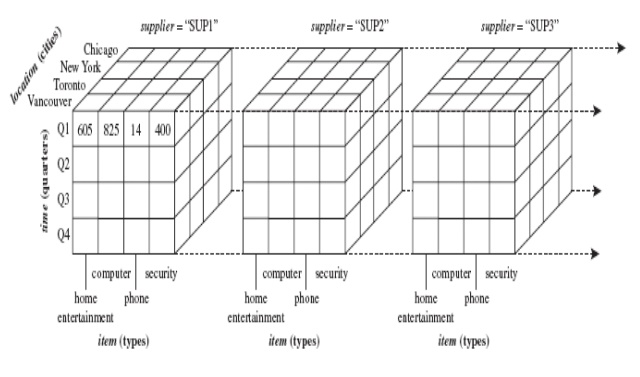Home | | Data Ware Housing and Data Mining | Multidimensional Data Model

# Multidimensional Data Model

The multidimensional data model is an integral part of On-Line Analytical Processing, or OLAP

Multidimensional Data Model

The multidimensional data model is an integral part of On-Line Analytical Processing, or OLAP

Multidimensional data model is to view it as a cube. The cable at the left contains detailed sales data by product, market and time. The cube on the right associates sales number (unit sold) with dimensions-product type, market and time with the unit variables organized as cell in an array.

This cube can be expended to include another array-price-which can be associates with all or only some dimensions. As number of dimensions increases number of cubes cell increase exponentially.

Dimensions are hierarchical in nature i.e. time dimension may contain hierarchies for years, quarters, months, weak and day. GEOGRAPHY may contain country, state, city etc.Star schema: A fact table in the middle connected to a set of dimension tables It contains:

A large central table (fact table)

A set of smaller attendant tables (dimension table), one for each dimensionSnowflake schema: A refinement of star schema where some dimensional hierarchy is further splitting (normalized) into a set of smaller dimension tables, forming a shape similar to snowflake

However, the snowflake structure can reduce the effectiveness of browsing, since more joins will be neededFact constellations: Multiple fact tables share dimension tables, viewed as a collection of stars, therefore called galaxy schema or fact constellationIn this cube we can observe, that each side of the cube represents one of the elements of the question. The x-axis represents the time, the y-axis represents the products and the z-axis represents different centers. The cells of in the cube represents the number of product sold or can represent the price of the items.

This Figure also gives a different understanding to the drilling down operations. The relations defined must not be directly related, they related directly.

The size of the dimension increase, the size of the cube will also increase exponentially. The time response of the cube depends on the size of the cube.

Operations in Multidimensional Data Model:

Aggregation (roll-up)

dimension reduction: e.g., total sales by city

summarization over aggregate hierarchy: e.g., total sales by city and year -> total sales by region and by year

Selection (slice) defines a subcube

e.g., sales where city = Palo Alto and date = 1/15/96